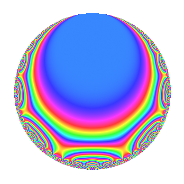# Properties

 Label 672.2.h.dLevel 672 Weight 2 Character orbit 672.h Analytic conductor 5.366 Analytic rank 0 Dimension 4 CM No Inner twists 2

# Related objects

## Newspace parameters

 Level: $$N$$ = $$672 = 2^{5} \cdot 3 \cdot 7$$ Weight: $$k$$ = $$2$$ Character orbit: $$[\chi]$$ = 672.h (of order $$2$$ and degree $$1$$)

## Newform invariants

 Self dual: No Analytic conductor: $$5.36594701583$$ Analytic rank: $$0$$ Dimension: $$4$$ Coefficient field: $$\Q(\zeta_{8})$$ Coefficient ring: $$\Z[a_1, \ldots, a_{5}]$$ Coefficient ring index: $$1$$ Sato-Tate group: $\mathrm{SU}(2)[C_{2}]$

## $q$-expansion

Coefficients of the $$q$$-expansion are expressed in terms of a primitive root of unity $$\zeta_{8}$$. We also show the integral $$q$$-expansion of the trace form.

 $$f(q)$$ $$=$$ $$q + ( 1 + \zeta_{8}^{2} - \zeta_{8}^{3} ) q^{3} + ( -\zeta_{8} + 2 \zeta_{8}^{2} - \zeta_{8}^{3} ) q^{5} + \zeta_{8}^{2} q^{7} + ( 2 \zeta_{8} + \zeta_{8}^{2} - 2 \zeta_{8}^{3} ) q^{9} +O(q^{10})$$ $$q + ( 1 + \zeta_{8}^{2} - \zeta_{8}^{3} ) q^{3} + ( -\zeta_{8} + 2 \zeta_{8}^{2} - \zeta_{8}^{3} ) q^{5} + \zeta_{8}^{2} q^{7} + ( 2 \zeta_{8} + \zeta_{8}^{2} - 2 \zeta_{8}^{3} ) q^{9} + 2 q^{11} + ( 2 - \zeta_{8} + \zeta_{8}^{3} ) q^{13} + ( -3 + 2 \zeta_{8} + \zeta_{8}^{2} - 2 \zeta_{8}^{3} ) q^{15} + ( -2 \zeta_{8} + 2 \zeta_{8}^{2} - 2 \zeta_{8}^{3} ) q^{17} + ( 3 \zeta_{8} - 2 \zeta_{8}^{2} + 3 \zeta_{8}^{3} ) q^{19} + ( -1 + \zeta_{8} + \zeta_{8}^{2} ) q^{21} -4 q^{23} + ( -1 + 4 \zeta_{8} - 4 \zeta_{8}^{3} ) q^{25} + ( 1 + 5 \zeta_{8} - \zeta_{8}^{2} ) q^{27} + ( -2 \zeta_{8} + 2 \zeta_{8}^{2} - 2 \zeta_{8}^{3} ) q^{29} + ( 2 \zeta_{8} + 4 \zeta_{8}^{2} + 2 \zeta_{8}^{3} ) q^{31} + ( 2 + 2 \zeta_{8}^{2} - 2 \zeta_{8}^{3} ) q^{33} + ( -2 + \zeta_{8} - \zeta_{8}^{3} ) q^{35} + ( 2 + 2 \zeta_{8} - 2 \zeta_{8}^{3} ) q^{37} + ( 1 - 2 \zeta_{8} + 3 \zeta_{8}^{2} - 2 \zeta_{8}^{3} ) q^{39} -10 \zeta_{8}^{2} q^{41} + ( 6 \zeta_{8} - 2 \zeta_{8}^{2} + 6 \zeta_{8}^{3} ) q^{43} + ( -2 + 5 \zeta_{8} - 4 \zeta_{8}^{2} + 3 \zeta_{8}^{3} ) q^{45} + ( -4 - 4 \zeta_{8} + 4 \zeta_{8}^{3} ) q^{47} - q^{49} + ( -4 + 2 \zeta_{8} - 4 \zeta_{8}^{3} ) q^{51} + ( -8 \zeta_{8} + 2 \zeta_{8}^{2} - 8 \zeta_{8}^{3} ) q^{53} + ( -2 \zeta_{8} + 4 \zeta_{8}^{2} - 2 \zeta_{8}^{3} ) q^{55} + ( 5 - 2 \zeta_{8} + \zeta_{8}^{2} + 6 \zeta_{8}^{3} ) q^{57} + ( -2 + 3 \zeta_{8} - 3 \zeta_{8}^{3} ) q^{59} + ( 10 - 3 \zeta_{8} + 3 \zeta_{8}^{3} ) q^{61} + ( -1 + 2 \zeta_{8} + 2 \zeta_{8}^{3} ) q^{63} + ( -4 \zeta_{8} + 6 \zeta_{8}^{2} - 4 \zeta_{8}^{3} ) q^{65} + ( -8 \zeta_{8} - 2 \zeta_{8}^{2} - 8 \zeta_{8}^{3} ) q^{67} + ( -4 - 4 \zeta_{8}^{2} + 4 \zeta_{8}^{3} ) q^{69} + ( -6 - 4 \zeta_{8} + 4 \zeta_{8}^{3} ) q^{71} + ( -6 - 2 \zeta_{8} + 2 \zeta_{8}^{3} ) q^{73} + ( 3 + 8 \zeta_{8} - 5 \zeta_{8}^{2} + \zeta_{8}^{3} ) q^{75} + 2 \zeta_{8}^{2} q^{77} + 4 \zeta_{8}^{2} q^{79} + ( 7 + 4 \zeta_{8} + 4 \zeta_{8}^{3} ) q^{81} + ( 10 - \zeta_{8} + \zeta_{8}^{3} ) q^{83} + ( -8 + 6 \zeta_{8} - 6 \zeta_{8}^{3} ) q^{85} + ( -4 + 2 \zeta_{8} - 4 \zeta_{8}^{3} ) q^{87} + ( -4 \zeta_{8} + 2 \zeta_{8}^{2} - 4 \zeta_{8}^{3} ) q^{89} + ( -\zeta_{8} + 2 \zeta_{8}^{2} - \zeta_{8}^{3} ) q^{91} + ( -2 + 4 \zeta_{8} + 6 \zeta_{8}^{2} + 4 \zeta_{8}^{3} ) q^{93} + ( 10 - 8 \zeta_{8} + 8 \zeta_{8}^{3} ) q^{95} + ( -6 - 8 \zeta_{8} + 8 \zeta_{8}^{3} ) q^{97} + ( 4 \zeta_{8} + 2 \zeta_{8}^{2} - 4 \zeta_{8}^{3} ) q^{99} +O(q^{100})$$ $$\operatorname{Tr}(f)(q)$$ $$=$$ $$4q + 4q^{3} + O(q^{10})$$ $$4q + 4q^{3} + 8q^{11} + 8q^{13} - 12q^{15} - 4q^{21} - 16q^{23} - 4q^{25} + 4q^{27} + 8q^{33} - 8q^{35} + 8q^{37} + 4q^{39} - 8q^{45} - 16q^{47} - 4q^{49} - 16q^{51} + 20q^{57} - 8q^{59} + 40q^{61} - 4q^{63} - 16q^{69} - 24q^{71} - 24q^{73} + 12q^{75} + 28q^{81} + 40q^{83} - 32q^{85} - 16q^{87} - 8q^{93} + 40q^{95} - 24q^{97} + O(q^{100})$$

## Character Values

We give the values of $$\chi$$ on generators for $$\left(\mathbb{Z}/672\mathbb{Z}\right)^\times$$.

 $$n$$ $$127$$ $$421$$ $$449$$ $$577$$ $$\chi(n)$$ $$-1$$ $$1$$ $$-1$$ $$1$$

## Embeddings

For each embedding $$\iota_m$$ of the coefficient field, the values $$\iota_m(a_n)$$ are shown below.

For more information on an embedded modular form you can click on its label.

Label $$\iota_m(\nu)$$ $$a_{2}$$ $$a_{3}$$ $$a_{4}$$ $$a_{5}$$ $$a_{6}$$ $$a_{7}$$ $$a_{8}$$ $$a_{9}$$ $$a_{10}$$
575.1
 −0.707107 + 0.707107i −0.707107 − 0.707107i 0.707107 − 0.707107i 0.707107 + 0.707107i
0 0.292893 1.70711i 0 3.41421i 0 1.00000i 0 −2.82843 1.00000i 0
575.2 0 0.292893 + 1.70711i 0 3.41421i 0 1.00000i 0 −2.82843 + 1.00000i 0
575.3 0 1.70711 0.292893i 0 0.585786i 0 1.00000i 0 2.82843 1.00000i 0
575.4 0 1.70711 + 0.292893i 0 0.585786i 0 1.00000i 0 2.82843 + 1.00000i 0
 $$n$$: e.g. 2-40 or 990-1000 Significant digits: Format: Complex embeddings Normalized embeddings Satake parameters Satake angles

## Inner twists

Char. orbit Parity Mult. Self Twist Proved
1.a Even 1 trivial yes
12.b Even 1 yes

## Hecke kernels

This newform can be constructed as the intersection of the kernels of the following linear operators acting on $$S_{2}^{\mathrm{new}}(672, [\chi])$$:

 $$T_{5}^{4} + 12 T_{5}^{2} + 4$$ $$T_{11} - 2$$